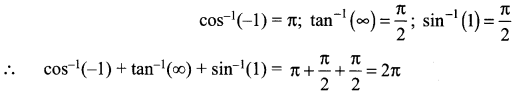# Samacheer Kalvi 12th Maths Solutions Chapter 4 Inverse Trigonometric Functions Ex 4.6

## Tamilnadu Samacheer Kalvi 12th Maths Solutions Chapter 4 Inverse Trigonometric Functions Ex 4.6

Choose the correct or the most suitable answer from the given four alternatives.
Question 1.
The value of sin-1(cos x), 0 ≤ x ≤ π is …………
(a) π – x
(b) x – $$\frac{\pi}{2}$$
(c) $$\frac{\pi}{2}$$ – x
(d) π – x
Solution:
(c) $$\frac{\pi}{2}$$ – x
Hint: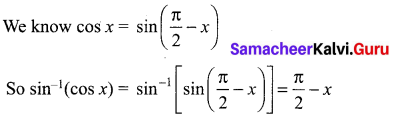Question 2.
If sin-1 + sin-1 y = $$\frac{2 \pi}{3}$$; then cos-1x + cos-1y is equal to ………….
(a) $$\frac{2 \pi}{3}$$
(b) $$\frac{\pi}{3}$$
(c) $$\frac{\pi}{6}$$
(d) π
Solution:
(b) $$\frac{\pi}{3}$$
Hint: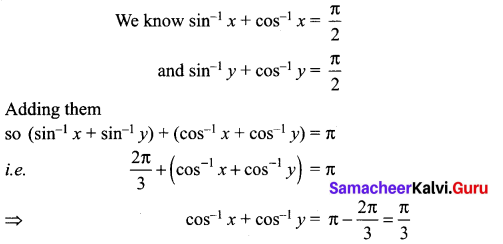Question 3.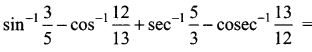……………
(a) 2π
(b) π
(c) 0
(d) tan-1$$\frac{12}{65}$$
Solution:
(c) 0
Hint: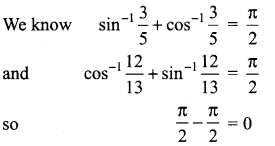Question 4.
If sin-1x = 2 sin-1 α has a solution, then ……………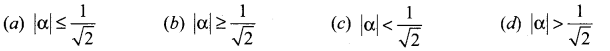Solution:
(a) |α| ≤ $$\frac{1}{\sqrt{2}}$$
Hint: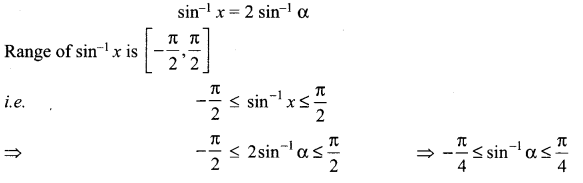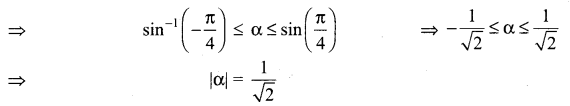Question 5.
sin-1 (cos x) = $$\frac{\pi}{2}$$ – x is valid for ……………..
(a) -π ≤ x ≤ 0
(b) 0 ≤ x ≤ π
(c) $$-\frac{\pi}{2}$$ ≤ x ≤ $$\frac{\pi}{2}$$
(d) $$-\frac{\pi}{4}$$ ≤ x ≤ $$\frac{3 \pi}{4}$$
Solution:
(b) 0 ≤ x ≤ π

Question 6.
If sin-1 x + sin-1 y + sin-1 z = $$\frac{3 \pi}{2}$$, the value of x2017 + y2018 + z2019 – $$\frac{9}{x^{101}+y^{101}+z^{101}}$$ is ……………….
(a) 0
(b) 1
(c) 2
(d) 3
Solution:
(a) 0
Hint:
The maximum value of sin-1 x is $$\frac{\pi}{2}$$ and sin-1 1 = $$\frac{\pi}{2}$$
Here it is given that
sin-1 x + sin-1 y + sin-1 z = $$\frac{3 \pi}{2}$$
⇒ x = y = z = 1
and so 1 + 1 + 1 – $$\frac{9}{1+1+1}$$ = 3 – 3 = 0

Question 7.
If cot-1 x = $$\frac{2 \pi}{5}$$ for some x ∈ R, the value of tan-1 x is …………
(a) $$-\frac{\pi}{10}$$
(b) $$\frac{\pi}{5}$$
(c) $$\frac{\pi}{10}$$
(d) $$-\frac{\pi}{5}$$
Solution:
(c) $$\frac{\pi}{10}$$
Hint: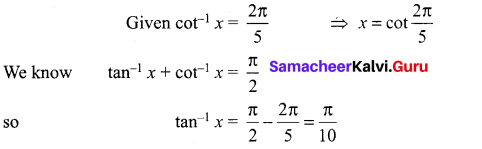Question 8.
The domain of the function defined by f(x) = sin-1 $$\sqrt{x-1}$$ is …………….
(a) [1, 2]
(b) [-1, 1]
(c) [0, 1]
(d) [-1, 0]
Solution:
(a) [1, 2]
Hint:
The domain for sin-1 x is [0, 1]
So $$\sqrt{x-1}$$ = 0 ⇒ x – 1 = 0 ⇒ x = 1
$$\sqrt{x-1}$$ = 1 ⇒ x – 1 = 0 ⇒ x = 2
∴ The domain is [1, 2]Question 9.
If x = $$\frac{1}{5}$$, the value of cos(cos-1 x + 2 sin-1 x) is …………….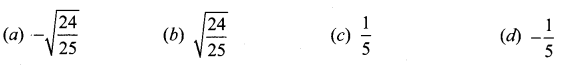Solution:
(d) $$-\frac{1}{5}$$
Hint: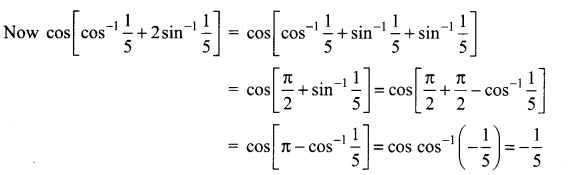Question 10.
$$\tan ^{-1}\left(\frac{1}{4}\right)+\tan ^{-1}\left(\frac{2}{9}\right)$$ is equal to …………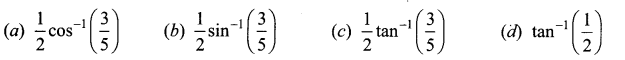Solution:
(d) tan-1$$\frac{1}{2}$$
Hint: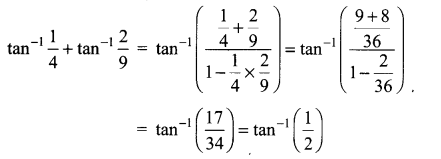Question 11.
If the function f(x) = sin-1(x2 – 3), then x belongs to …………..
(a) [1, -1]
(b) [$$\sqrt{2}$$, 2]
(c) $$[-2,-\sqrt{2}] \cup[\sqrt{2}, 2]$$
(d) $$[-2,-\sqrt{2}] \cap[\sqrt{2}, 2]$$
Solution:
(c) $$[-2,-\sqrt{2}] \cup[\sqrt{2}, 2]$$
Hint:
f(x) = sin-1(x2 – 3)
Domain of sin-1 (x) is [-1, 1]
⇒ -1 ≤ x2 – 3 ≤ 1 ⇒ 2 ≤ x2 ≤ 4
⇒ $$\sqrt{2}$$ ≤ x ≤ 2 ⇒ $$\sqrt{2}$$ ≤ |x| ≤ 2
x ∈ $$[-2,-\sqrt{2}] \cup[\sqrt{2}, 2]$$

Question 12.
If cot-1 2 and cot-1 3 are two angles of a triangle, then the third angle is …………..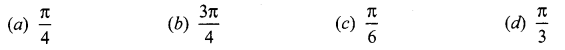Solution:
(b) $$\frac{3 \pi}{4}$$
Hint: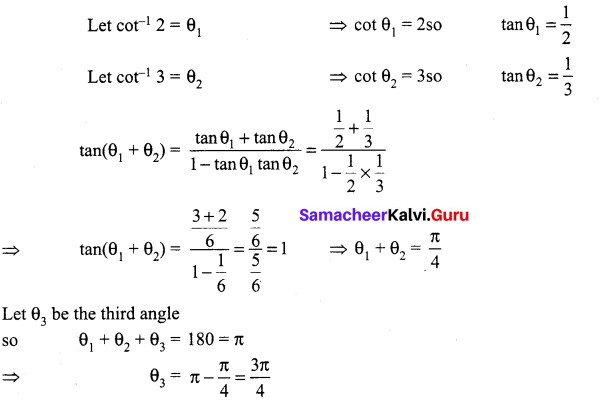Question 13.
$$\sin ^{-1}\left(\tan \frac{\pi}{4}\right)-\sin ^{-1}(\sqrt{\frac{3}{x}})=\frac{\pi}{6}$$. Then x is a root of the equation …………..
(a) x2 – x – 6 = 0
(b) x2 – x – 12 = 0
(c) x2 + x – 12 = 0
(d) x2 + x – 6 = 0
Solution:
(b) x2 – x – 12 = 0
Hint: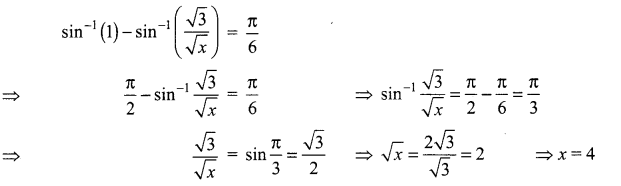Question 14.
sin-1(2 cos2 x – 1) + cos-1(1 – 2 sin2 x) = ……………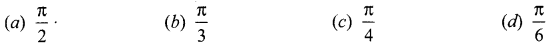Solution:
(a) $$\frac{\pi}{2}$$
Hint:
2 cos2x – 1 = cos 2x
1 – 2 sin2 x = cos 2x
∴ sin-1 x(cos 2x) + cos-1(cos 2x) = $$\frac{\pi}{2}$$ (∵ sin-1 x + cos-1 x = $$\frac{\pi}{2}$$)

Question 15.
If $$\cot ^{-1}(\sqrt{\sin \alpha})+\tan ^{-1}(\sqrt{\sin \alpha})$$ = u, then cos 2u is equal to …………..
(a) tan2 α
(b) 0
(c) -1
(d) tan 2α
Solution:
(c) -1
Hint:
cot-1 x + tan-1 x = $$\frac{\pi}{2}$$ ⇒ u = $$\frac{\pi}{2}$$ so 2u = π
∴ cos 2u = cos π = -1

Question 16.
If |x| ≤ 1, then 2tan-1 x – sin-1 $$\frac{2 x}{1+x^{2}}$$ is equal to ………….
(a) tan-1 x
(b) sin-1 x
(c) 0
(d) π
Solution:
(c) 0
Hint:
Let x = tan θ so $$\frac{2 x}{1+x^{2}}$$ = sin 2θ.
Now 2 tan-1(tanθ) – sin-1(sin 2θ) = 2θ – 2θ = 0

Question 17.
The equation tan-1 x – cot-1 x = tan-1 $$\left(\frac{1}{\sqrt{3}}\right)$$ has …………..
(a) no solution
(b) unique solution
(c) two solutions
(d) infinite number of solutions
Solution:
(b) unique solution
Hint: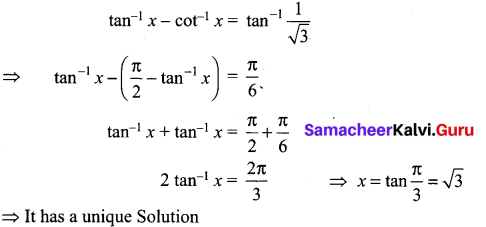Question 18.
If sin-1 x + cot-1 $$\left(\frac{1}{2}\right)=\frac{\pi}{2}$$, then x is equal to …………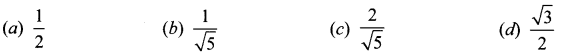Solution:
(b) $$\frac{1}{\sqrt{5}}$$
Hint:
sin-1 x + cot-1 $$\left(\frac{1}{2}\right)=\frac{\pi}{2}$$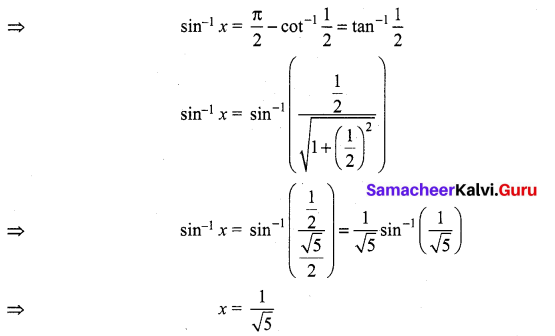Question 19.
If sin-1$$\frac{x}{5}$$ + cosec-1$$\frac{5}{4}$$ = $$\frac{\pi}{2}$$, then the value of x is ………
(a) 4
(b) 5
(c) 2
(d) 3
Solution:
(d) 3
Hint: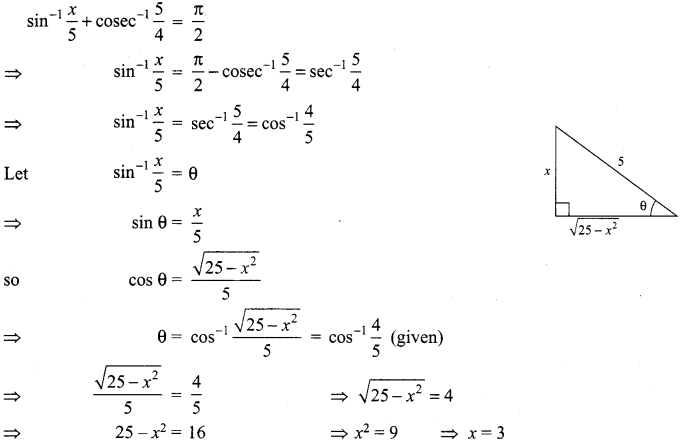Question 20.
sin(tan-1), |x| < 1 is equal to ………….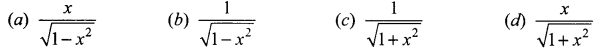Solution:
(d) $$\frac{x}{\sqrt{1+x^{2}}}$$
Hint: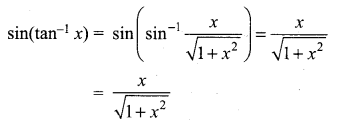### Samacheer Kalvi 12th Maths Solutions Chapter 4 Inverse Trigonometric Functions Ex 4.6 Additional Questions

Question 1.
Find the principal value of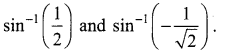Solution: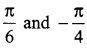Question 2.
Find the principal value of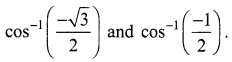Solution: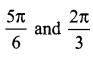Question 3.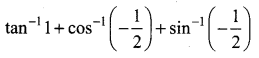Solution: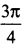Question 4.
Evaluate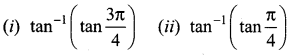Solution: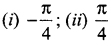Question 5.
Evaluate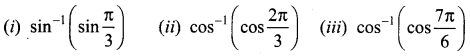Solution: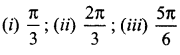Question 6.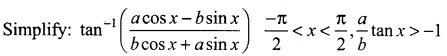Question 7.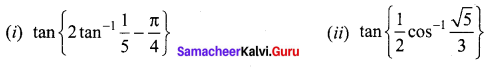Solution: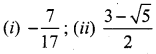Question 8.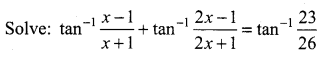Solution:
x = $$\frac{1}{6}$$

Question 9.
Find the values of each of the following: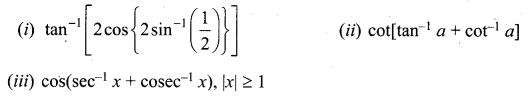Solution: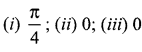Question 10.
Solve for x: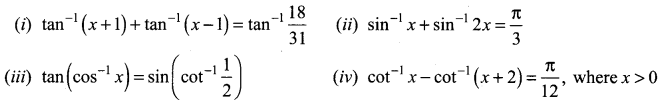Solution: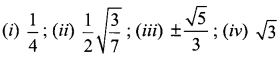Question 11.
Prove: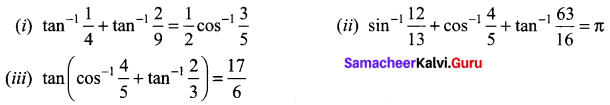Question 12.
Evaluate: sin(tan-1 x + cot-1 x)

Question 13.
The value of sin-1(1) + sin-1(0) is …….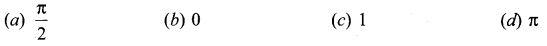Solution:
(a) $$\frac{\pi}{2}$$
Hint: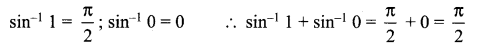Question 14.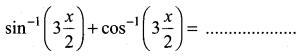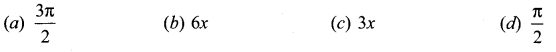Solution:
(d) $$\frac{\pi}{2}$$
Hint: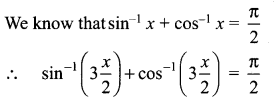Question 15.
tan-1x + cot-1x = ……..
(a) 1
(b) – π
(c) $$\frac{\pi}{2}$$
(d) π
Solution:
(c) $$\frac{\pi}{2}$$
Hint: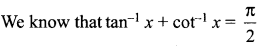Question 16.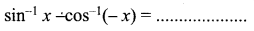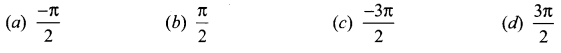Solution:
(a) $$\frac{-\pi}{2}$$
Hint: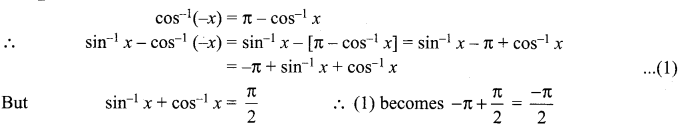Question 17.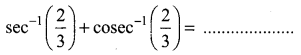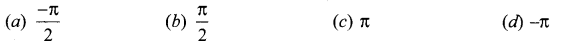Solution:
(b) $$\frac{\pi}{2}$$
Hint: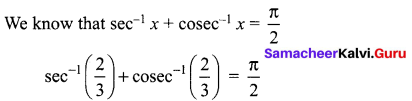Question 18.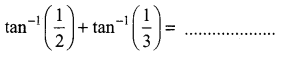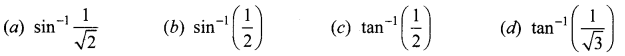Solution:
(a) $$\sin ^{-1} \frac{1}{\sqrt{2}}$$
Hint: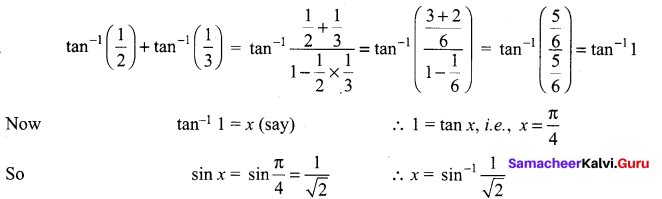Question 19.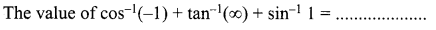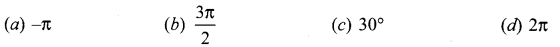Solution:
(d) 2π
Hint: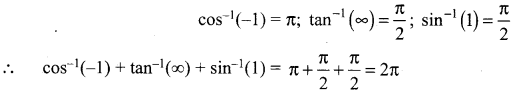Question 20.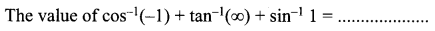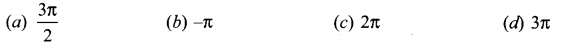Solution:
(c) 2π
Hint: